Question Pool Trigonometry

# Trigonometry - SAMAGRA Question Pool & Answers | Class 10 English Medium

Kerala Syllabus SAMAGRA SCERT SAMAGRA Question Pool for Class 10 English Medium Maths TrigonometryQn 1.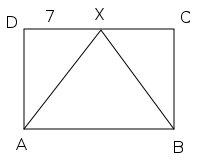In the figure X is the mid point of DC.. ?AXB is an equilateral triangle and ABCD is a rectangle also DX = 7 cm

a) What is the measure of∠ AXB?

b) What is the measure of∠ DAX ?

c) Calculate the area of the rectangle.

Get Free Study Materials + 1 Week Free Trial of BrainsPrep Class 10 English Medium Tuition

Qn 2.

In the figure the radius of the circle is 6cm,AB=AC and ∠ B=70°.

Find

a) ∠ A

b) The length of BC ?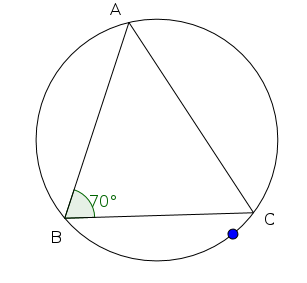Angle sin cos tan 40° .6428 .7660 .8391 70° .9397 .3420 2.7475

a) ∠ A=40°

b)

Get Free Study Materials + 1 Week Free Trial of BrainsPrep Class 10 English Medium Tuition

Qn 3.

In ?ABC , BC= 3cm, ∠ B=45°, ∠ C=75°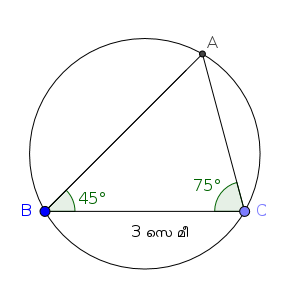a) Find ∠ A?

b) Find the circum radius ?

Get Free Study Materials + 1 Week Free Trial of BrainsPrep Class 10 English Medium Tuition

Qn 4.

One of the angles of the right angled triangle ABC is 45° .The length of one of the perpendicular sides is 1 unit.

a) Find the value of sin45°

b) Prove that tan 45° =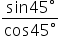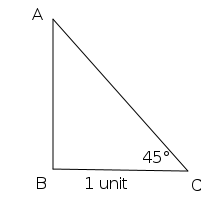Get Free Study Materials + 1 Week Free Trial of BrainsPrep Class 10 English Medium Tuition

Qn 5.

a) write the ratio of the sides of the triangle in the figure.

b) Which is the smallest angle in this triangle.? What is its measure?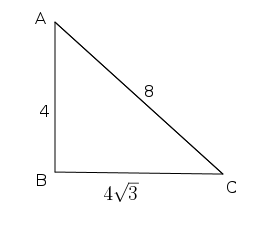4  : 4√3  : 8  =1: √3 : 2

Get Free Study Materials + 1 Week Free Trial of BrainsPrep Class 10 English Medium Tuition

Qn 6.

In ? ABC , BC=5 cm and ∠ C=40°

Find the length of AB?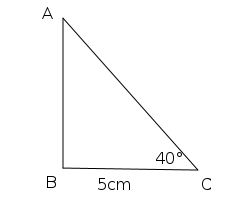????‍ sin cos tan 40° .6428 .7660 .8391 50° .7660 .6428 1.1918

Get Free Study Materials + 1 Week Free Trial of BrainsPrep Class 10 English Medium Tuition

Qn 7.

In the figure the radius of the circle is 5 cm . ∠ AOB= 90° . then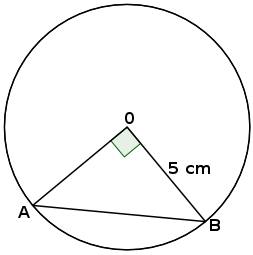1. Find the length of AB.

2.Calculate the area of the triangle.

Get Free Study Materials + 1 Week Free Trial of BrainsPrep Class 10 English Medium Tuition

Qn 8.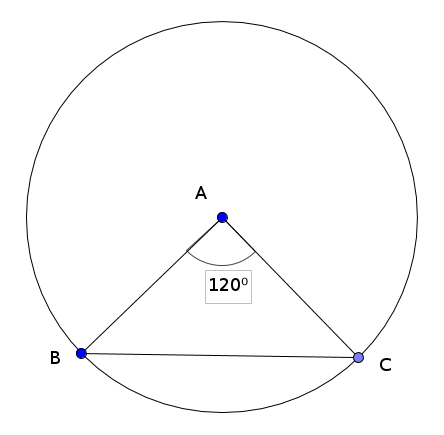In the figure A is the centre of the circle and ∠A=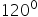a) Find AB : AC : BC

b) If AB = 8 cm what is length of BC ?

Get Free Study Materials + 1 Week Free Trial of BrainsPrep Class 10 English Medium Tuition

Qn 9.

In triangle ABC , ∠ B=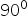, AC = 10 cm , BC = 6 cm . Find Sin A and Cos A.

Get Free Study Materials + 1 Week Free Trial of BrainsPrep Class 10 English Medium Tuition

Qn 10.

Find the radius of the circle.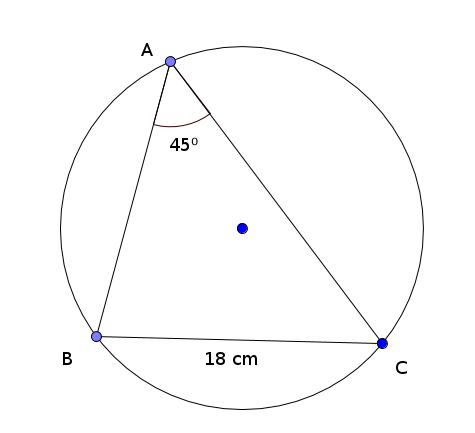Get Free Study Materials + 1 Week Free Trial of BrainsPrep Class 10 English Medium Tuition

Qn 11.

The diagonal of a rectangle is 16 centimetres. This diagonal makes an angle 30° with one side of the rectangle.

a) Find the length and breadth of the rectangle?

b) What is the area of the rectangle ?

Get Free Study Materials + 1 Week Free Trial of BrainsPrep Class 10 English Medium Tuition

Qn 12.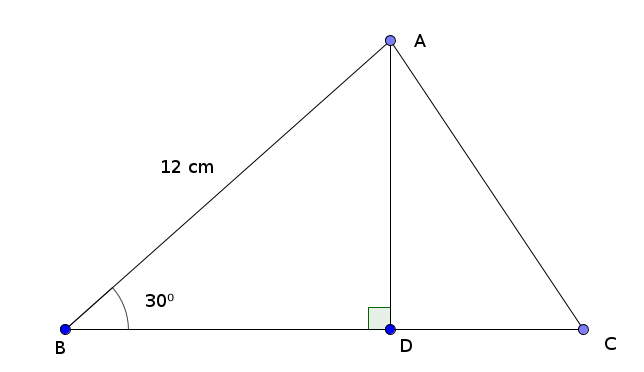In the figure AB = 12 cm , ∠B=30°

a) What is the length of AD ?

b) If BC = 15 cm , Find the area of the triangle.

a) Identifying the angles of the triangle as 30°, 60°, 90°

Get Free Study Materials + 1 Week Free Trial of BrainsPrep Class 10 English Medium Tuition

Qn 13.

Angle    sin                cos

0           0.0000        1.0000

1           0.0175        0.9998

2           0.0349        0.9994

3           0.0523        0.9986

.

.

.

.

87           0.9986        0.0523

88           0.9994        0.0349

89           0.9998        0.0175

90           1.0000        0.0000

Observing the table we have sin 0 = cos 90 = 0.0000 , sin 1 = cos 89 = 0.0175
sin 2 = cos 88 = 0.0349 ....... Then answer the questions given below.

a) What is the value of sin 90 ?

b) If sin 10 = cos p , what is the value of p ?

c) Find the value of x which satisfies sin x = cos x

d) If sin x = cos y ,then  x+y = ..........

e) Arrange sin 5, cos 5, sin 10 in ascending order of values.

a) sin 90 = 1 or cos 0

b) p = 80

Get Free Study Materials + 1 Week Free Trial of BrainsPrep Class 10 English Medium Tuition

Qn 14.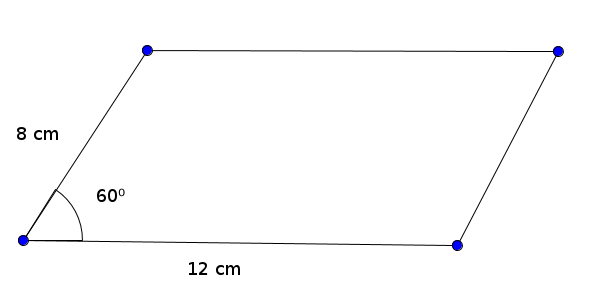In the figure two sides of the parallelogram are 8 cm , 12 cm and the angle between these sides is 60°.

a) What is the distance between the lengths ?

b) What is the area of the parallelogram ?

Get Free Study Materials + 1 Week Free Trial of BrainsPrep Class 10 English Medium Tuition

Qn 15.

a) What is the ratio of the sides of a triangle with angles 45°, 45°, 90° ?

b) What is the length of the hypotenuse of such a triangle if the opposite side of angle 45° is 5 centimetre ?

Get Free Study Materials + 1 Week Free Trial of BrainsPrep Class 10 English Medium Tuition

Qn 16.

A 1.5 meter tall boy sees the top of a 15 meter high building at an angle of elevation  50°.

a) Based on these facts draw a rough figure.

b) Find the distance between the boy and the building?

(sin 50°= 0.7660 cos50°= 0.6428 tan 50°= 1.1918)

Get Free Study Materials + 1 Week Free Trial of BrainsPrep Class 10 English Medium Tuition

Qn 17.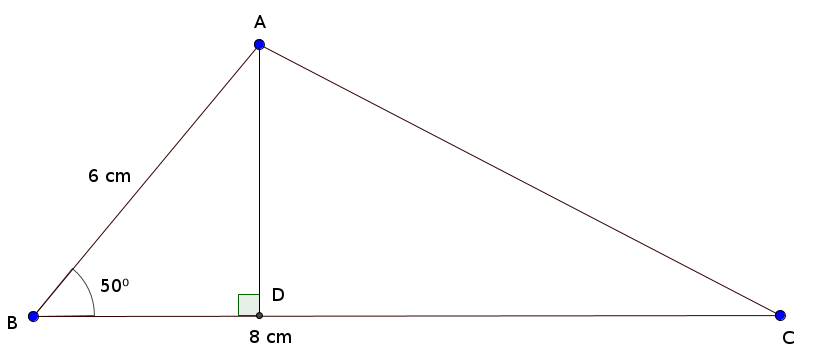a) Draw the figure with given measures.

c) Find the length of AD using trigonometry.

(sin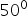=.77   cos=.64  tan=  1 .2 )

a) For Drawing figure

Get Free Study Materials + 1 Week Free Trial of BrainsPrep Class 10 English Medium Tuition

Qn 18.

From the top of a tower of height 10 m , a car on the ground was seen at an angle of depression 25°.

a) Draw a rough figure.

b) Find the distance between the car and the tower?

Get Free Study Materials + 1 Week Free Trial of BrainsPrep Class 10 English Medium Tuition

Qn 19.

The vertices of a regular pentagon are on the circle of radius 10 cm.

1.Find ∠ COD?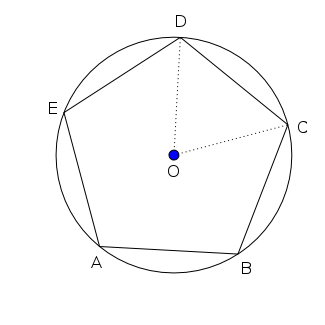2. Calculate the perimeter of the pentagon?

(Sin36= 0.5878 , cos36=0.8090 , tan36= =0.7265)

a) ∠ COD=72°

Get Free Study Materials + 1 Week Free Trial of BrainsPrep Class 10 English Medium Tuition

Qn 20.

In the figure O is the centre of the circle ,BE= 5cm, BC= 6cm ∠ EAB=40°.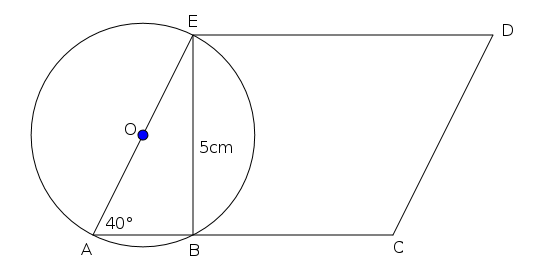a) Find ∠ ABE ?

b) What is the length of AB?

c) Calculate the area of the parallelogram ACDE

(Sin40°=.6428 cos40°=.7660

tan 40°=.8391)

(sin 50°=.7660 cos50°=.6428

tan 50°=1.1918)

a) ∠ ABE=90°

Get Free Study Materials + 1 Week Free Trial of BrainsPrep Class 10 English Medium Tuition

Qn 21.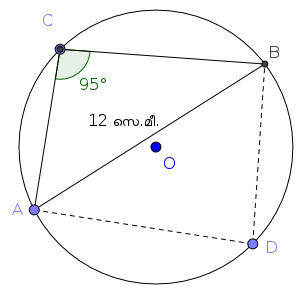In the figure , ∠ ACB= 95° and AB=12 cm,

b) Find the circum radius of ? ABC?

(sin 85°=.9962 cos 85°=.0872)

Get Free Study Materials + 1 Week Free Trial of BrainsPrep Class 10 English Medium Tuition

Qn 22.

In the figure ∠ P=90°, ∠ Q =35° ,and QR=12cm, Find

a) ∠ R

b) The length of PR

c) Area of ? PQR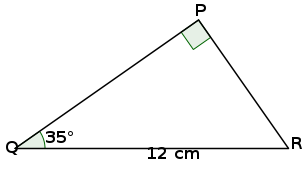Angle sin cos tan 35° .5736 .8192 .7002 55° .8192 .5736 1.4281

Get Free Study Materials + 1 Week Free Trial of BrainsPrep Class 10 English Medium Tuition

Qn 23.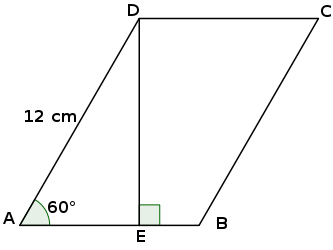In the parallelogram ABCD ,∠ A= 60° and AD=12cm.

a) Find the distance between the parallel lines AB and CD?

b) If AB= 9 cm, Calculate the area of the parallelogram

For finding the ratio oof the sides of the triangle 30° , 60° , 90° as  1:√3:2

Get Free Study Materials + 1 Week Free Trial of BrainsPrep Class 10 English Medium Tuition

Qn 24.

Find the circum diametre of an equilateral triangle with sides 18 cm, correct to two decimal places.

Get Free Study Materials + 1 Week Free Trial of BrainsPrep Class 10 English Medium Tuition

Qn 25.

A man standing on the ground sees the top of a 20 metre high building at an angle of elevation 450 . He sees the top of a mobile tower fixed on the building at an angle of elevation 600

a) Draw a rough figure based on these statements.

b) At what distance the man stands from the bottom of the building ?

c) Find the height of the tower.

Get Free Study Materials + 1 Week Free Trial of BrainsPrep Class 10 English Medium Tuition

Qn 26.

Two sides of a triangle are 9 cm , 8 cm and the angle between them is 700 . Find the area of the triangle.

(sin 700 = 0.9397, cos 700 = 0.3420, tan 700 = 2.7465)

Get Free Study Materials + 1 Week Free Trial of BrainsPrep Class 10 English Medium Tuition

Qn 27.

One angle of a triangle is 140° and its opposite side is 8 cm. What is the radius of its circumcircle ?

(sin 40° = 0.64 , cos 40° = 0.76 , tan 40°= 0.80)

Get Free Study Materials + 1 Week Free Trial of BrainsPrep Class 10 English Medium Tuition

Qn 28.

In the figure PQ = 10 cm , QR = 8 cm , ∠ Q = 1350

Find the area of the triangle.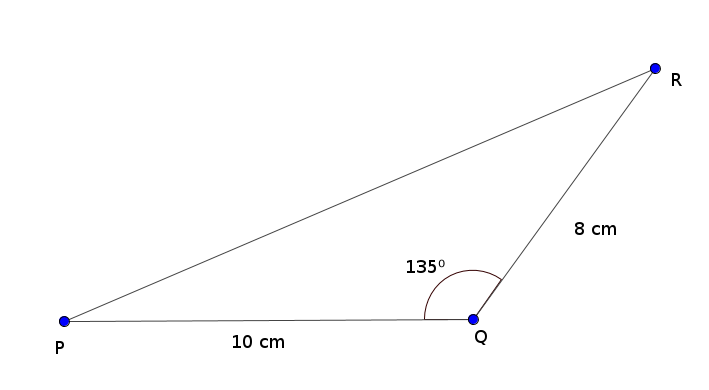Get Free Study Materials + 1 Week Free Trial of BrainsPrep Class 10 English Medium Tuition

Qn 29.

A pole erected perpendicular to the ground, and two ropes are fastened from the top of the pole to the ground, on either side of the pole. One rope makes an angle 500 with the ground. This rope touches the ground at a distance of 10 m from the foot of the pole. The other rope makes an angle 550 with the ground.

1. Draw a rough figure

2. What is the height of the pole?

3. What is the approximate length of the rope?

(sin50=0.77, cos50=0.64, tan50=1.19)

Get Free Study Materials + 1 Week Free Trial of BrainsPrep Class 10 English Medium Tuition